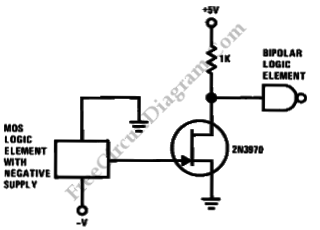## Precision Current Sink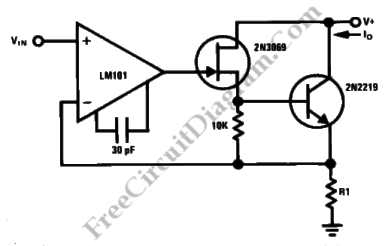This a a simple circuit for precision current sink. This circuit uses two kinds of transistor, 2N2219 bipolar transistor and 2N3069 JFET because both of them have inherently high output impedance. Here is the circuit : To provide feedback to the LM101 op amp, this circuit uses R1 as a current sensing resistor. The true current sink nature of this […]

## AC Mode Universal Motor Drive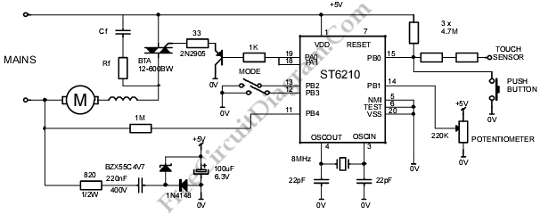This is AC mode universal motor drive circuit. This circuit is suitable for large range of application. It uses the microcontroller to interpret the user interface that is used to select the four operating modes that are defined in hardware. Here is the circuit : As power switch, this circuit use “SNUBBERLESS” TRIAC  BTA 12-600BW  with a maximum specified gate […]

## Soft Light Dimmer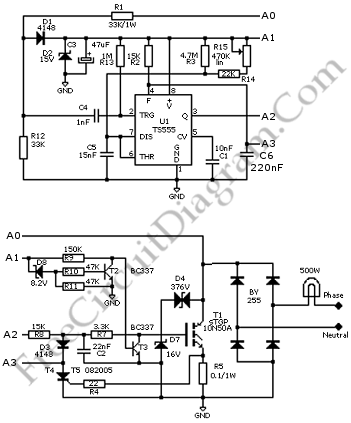This is circuit of soft light dimmer. This circuit uses the IGBT STGP10N50A and the TS555 timer as main components. Here is the circuit: The timer is triggered on the zero crossing voltage pulse. The time constant determined by C5/R14+R15 that is used to determine the conduction angle. The T2 – T3 inhibits the power switch until the auxiliary supply […]

## Temperature-Compensated Capacitive Proximity Detector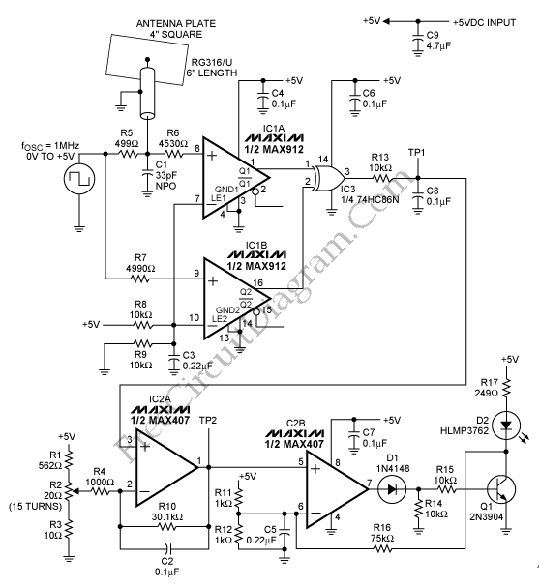This is a circuit of Temperature-Compensated Capacitive Proximity Detector. it will lights the LED when a person approaches the antenna plate. Here is the circuit : The 4-inch-square antenna plate and a 33pF capacitor (C1) in parallel with 15pF (6 inches of coaxial cable at 30pF per foot)  are used to form delay capacitance of this circuit. It charges to […]

## Half Supply Generator Provides Virtual Grounding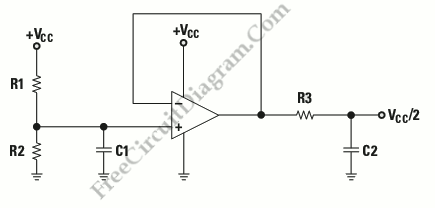This is a circuit that provide virtual ground that it is required on single-supply operation. This circuit consist of voltage divider and buffer. This circuit generates voltage equal to VCC/2. The voltage divider is bypassed by capacitor. This voltage is generated and buffered by the TLE2426. Here is the schematic diagram of half supply generator provides virtual grounding : To […]

## Voltage Controlled Variable Gain Amplifier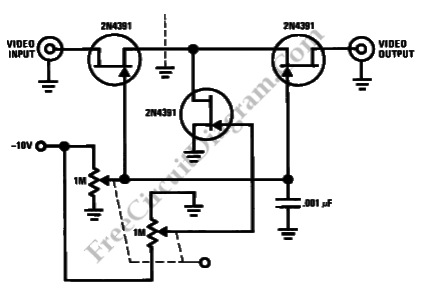This is a circuit of voltage controlled variable gain amplifier. This circuit uses 2N4391 that will provide a low Rds(on) less than 30 Ohm. This circuit also uses a tee attenuator, that will provide optimum dynamic linear range for attenuation. We can obtain the attenuation greater than 100dB at 10MHz by providing proper RF construction techniques. Here is the circuit […]